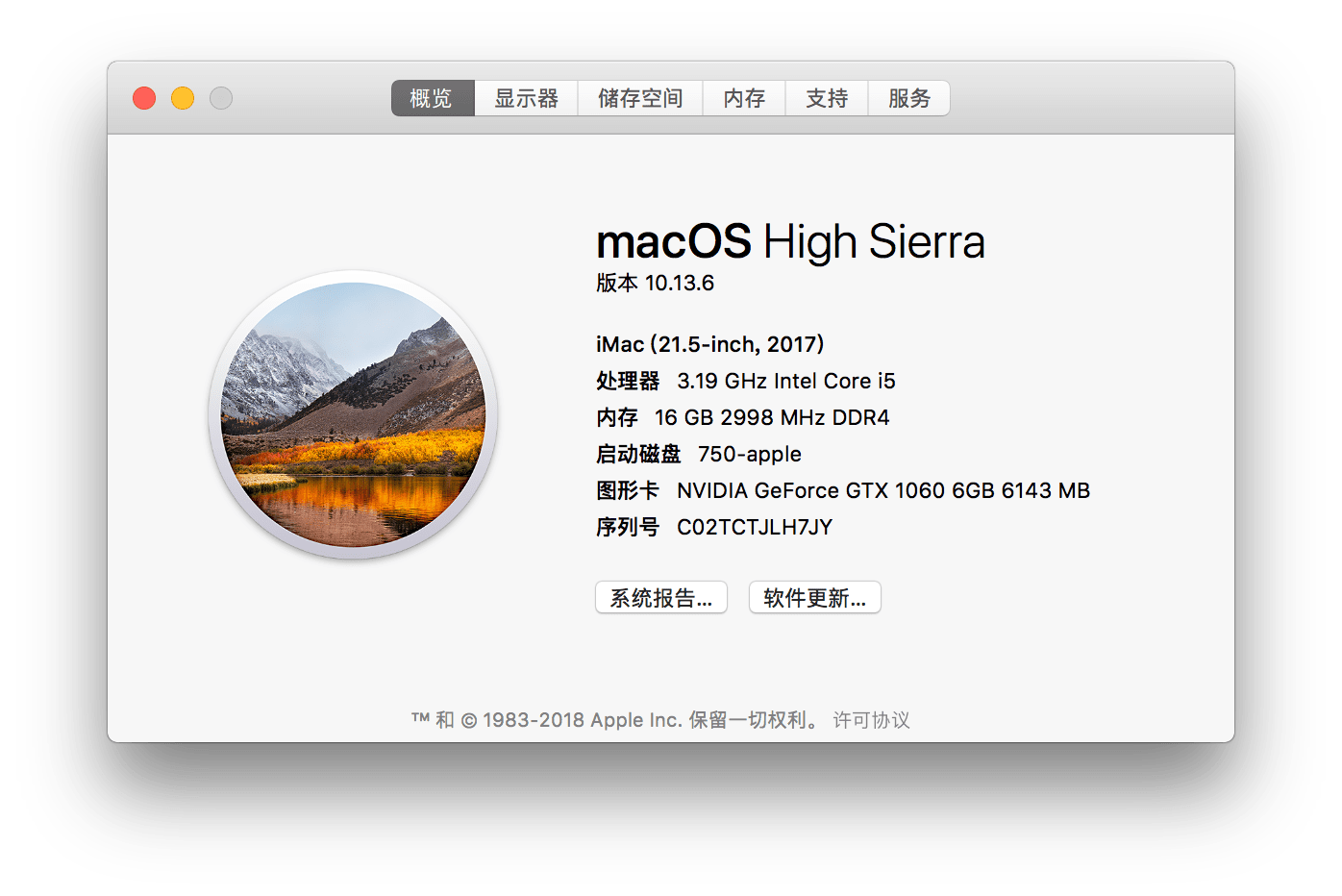- 阅读剩余部分 -

## 单循环链表

``````#!/usr/bin/env python3
# coding=utf-8

class Node:
def __init__(self, data):
self._data = data
self._next = None

def set_next(self, node):
self._next = node

def set_data(self, data):
self._data = data

def get_data(self):
return self._data

def get_next(self):
return self._next

def __init__(self):

# 清空/初始化
def clear(self):

# 是否为空
def is_empty(self):

# 获取大小
def size(self):
count = 0
count = count + 1
cur = cur.get_next()
return count

# 搜索是否存在
def search(self, data):
if cur.get_data() == data:
return True
cur = cur.get_next()
return False

# 移除指定元素
def remove(self, data):
cur = prev.get_next()
if cur.get_data() == data:
prev.set_next(cur.get_next())
prev = prev.get_next()

# 头插
def insert(self, data):
temp = Node(data)

# 尾插
def append(self, data):
# 建立node
temp = Node(data)
# 拿到最后一个node
cur = cur.get_next()
# 插入
cur.set_next(temp)

def print_me(self):
print cur.get_data()
cur = cur.get_next()

if __name__ == '__main__':
a.append('aa')
a.remove('aa')
a.print_me()``````

- 阅读剩余部分 -

## 记一次黑苹果的安装``````CPU: i56500

WIFI: BCM943602CS (黑苹果免驱，win10需要安装驱动)

- 阅读剩余部分 -

- 阅读剩余部分 -

- 阅读剩余部分 -